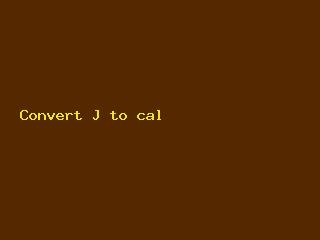Home Top Car Convert J to cal

# Convert J to cal

You Might Be Interested In

Rate this postPlease provide values below to convert joule [J] to calorie (th) [cal (th)], or vice versa.

## Joule

Definition: A joule (symbol: J) is a derived unit of energy in the International System of Units (SI). It is defined as the energy transferred to an object when a one newton force is applied to the object in the direction of its motion through a distance of one meter. It is also defined as the energy dissipated as heat when a 1 ampere electric current passes through a resistance of one ohm in the course of one second. It has a number of representations both in SI base units as well as other SI units such that:

J = kg·m2/s2 = N·m = Pa·m3 = W·s = C·V

History/origin: The unit, joule, is named after James Prescott Joule, an English physicist and mathematician who helped develop the Kelvin scale. He also discovered the relationship between heat and mechanical work, leading to the law of conservation of energy, and subsequently, the first law of thermodynamics.

Current use: As an SI derived unit, the joule is used within a variety of scientific contexts. Practical examples of energy measurement using joules include the energy required to lift objects, the energy released when objects fall, the heat required to raise temperature, and the kinetic energy of moving objects.

One of the dimensional representations of a joule is the N·m (Newton-meter), which is equivalent to the SI unit for torque. These units, however, are different and should be considered as such. Even though the joule is algebraically equal to the N·m, the N·m should not be used to represent the joule whenever possible to avoid confusion with torque.

## Calorie

Definition: A calorie (symbol: cal) is a unit of energy defined as the amount of energy required to increase the temperature of one gram of water by one °C. This is referred to as the small calorie or the gram calorie and is equal to 4.1868 joules, the SI (International System of Units) unit of energy.

A large calorie (symbol: Cal), also known as a kilogram calorie (symbol: Cal), is technically a kilocalorie (symbol: kcal), the equivalent of 1000 small calories but is also sometimes referred to as simply “Calorie.” Large calories are usually used for labeling foods and as such, is known as the food calorie.

History/origin: The term calorie comes from the Latin word “calor,” which means heat. It was first defined as a unit of heat energy in 1824 by Nicolas Clement and appeared in French and English dictionaries between 1841 and 1867. The large calorie was introduced to the American public later, in 1887, by Wilbur Olin Atwater.

READ:   10 Top Electric Cars for Under \$30,000 in 2023

Current use: Although the calorie can be used with SI, since the official adoption of SI in 1960, the calorie is considered obsolete. Despite this, the large calorie is still widely used as a unit of food energy, alongside, or in place of, the SI unit of food energy, the kilojoule. The use of a capital “C” in Calorie is intended to denote the use of kilocalories rather than calories denoting a single calorie. This is not often understood, however, resulting in some confusion when foods are only labeled as Calories rather than kilocalories.

Calories are also used within scientific contexts, such as chemistry. In these contexts, the term being referenced is most often the small calorie, though measurements are often reported in kilocalories.

## Joule to Calorie (th) Conversion Table

Joule [J] Calorie (th) [cal (th)]
0.01 J 0.0023900574 cal (th)
0.1 J 0.0239005736 cal (th)
1 J 0.2390057361 cal (th)
2 J 0.4780114723 cal (th)
3 J 0.7170172084 cal (th)
5 J 1.1950286807 cal (th)
10 J 2.3900573614 cal (th)
20 J 4.7801147228 cal (th)
50 J 11.9502868069 cal (th)
100 J 23.9005736138 cal (th)
1000 J 239.0057361377 cal (th)

## How to Convert Joule to Calorie (th)

1 J = 0.2390057361 cal (th)
1 cal (th) = 4.184 J

Example: convert 15 J to cal (th):
15 J = 15 × 0.2390057361 cal (th) = 3.5850860421 cal (th)